### Home > CC4 > Chapter 10 > Lesson 10.1.3 > Problem10-51

10-51.
1. Solve these equations, if possible. Each time, be sure you have found all possible solutions. Check your solutions. Homework Help ✎

1. (x + 4)2 = 49

2.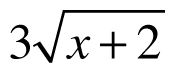= 12

3.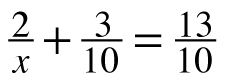4. 5(2x – 1) – 2 = 13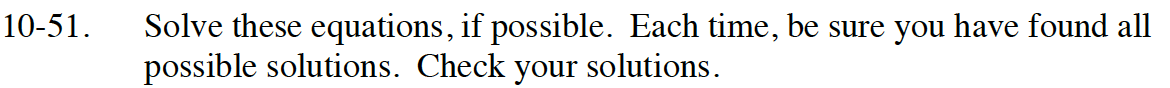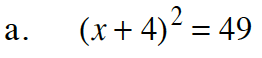$\sqrt{(\textit{x}+4)^2}=\sqrt{49}$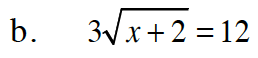Divide both sides by 3.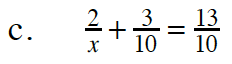$\frac{2}{\textit{x}}=\frac{10}{10}$

x = 2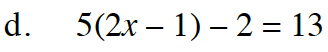Distribute, then solve.